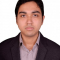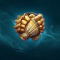Class Central is learner-supported. When you buy through links on our site, we may earn an affiliate commission.

# Differential Equations for Engineers

## Overview

##### Class Central Tips
This course is about differential equations and covers material that all engineers should know. Both basic theory and applications are taught. In the first five weeks we will learn about ordinary differential equations, and in the final week, partial differential equations.

The course is composed of 56 short lecture videos, with a few simple problems to solve following each lecture. And after each substantial topic, there is a short practice quiz. Solutions to the problems and practice quizzes can be found in instructor-provided lecture notes. There are a total of six weeks in the course, and at the end of each week there is an assessed quiz.

http://www.math.ust.hk/~machas/differential-equations-for-engineers.pdf

Watch the promotional video:
https://youtu.be/eSty7oo09ZI

## Syllabus

• First-Order Differential Equations
• A differential equation is an equation for a function with one or more of its derivatives. We introduce differential equations and classify them. We then learn about the Euler method for numerically solving a first-order ordinary differential equation (ode). Then we learn analytical methods for solving separable and linear first-order odes. An explanation of the theory is followed by illustrative solutions of some simple odes. Finally, we learn about three real-world examples of first-order odes: compound interest, terminal velocity of a falling mass, and the resistor-capacitor electrical circuit.
• Homogeneous Linear Differential Equations
• We generalize the Euler numerical method to a second-order ode. We then develop two theoretical concepts used for linear equations: the principle of superposition, and the Wronskian. Armed with these concepts, we can find analytical solutions to a homogeneous second-order ode with constant coefficients. We make use of an exponential ansatz, and transform the constant-coefficient ode to a quadratic equation called the characteristic equation of the ode. The characteristic equation may have real or complex roots and we learn solution methods for the different cases.
• Inhomogeneous Linear Differential Equations
• We now add an inhomogeneous term to the constant-coefficient ode. The inhomogeneous term may be an exponential, a sine or cosine, or a polynomial. We also study the phenomena of resonance, when the forcing frequency is equal to the natural frequency of the oscillator. Finally, we learn about three important applications: the RLC electrical circuit, a mass on a spring, and the pendulum.
• The Laplace Transform and Series Solution Methods
• We present two new analytical solution methods for solving linear odes. The first is the Laplace transform method, which is used to solve the constant-coefficient ode with a discontinuous or impulsive inhomogeneous term. The Laplace transform is a good vehicle in general for introducing sophisticated integral transform techniques within an easily understandable context. We also discuss the series solution of a linear ode. Although we do not go deeply here, an introduction to this technique may be useful to students that encounter it again in more advanced courses.
• Systems of Differential Equations
• We learn how to solve a coupled system of homogeneous first-order differential equations with constant coefficients. This system of odes can be written in matrix form, and we learn how to convert these equations into a standard matrix algebra eigenvalue problem. The two-dimensional solutions are visualized using phase portraits. We then learn about the important application of coupled harmonic oscillators and the calculation of normal modes. The normal modes are those motions for which the individual masses that make up the system oscillate with the same frequency.
• Partial Differential Equations
• To learn how to solve a partial differential equation (pde), we first define a Fourier series. We then derive the one-dimensional diffusion equation, which is a pde for the diffusion of a dye in a pipe. We proceed to solve this pde using the method of separation of variables.

### Taught by

Jeffrey R. Chasnov

## Reviews

4.9 rating, based on 252 reviews

Start your review of Differential Equations for Engineers

• Great course, great overall coverage of topics, application-based examples aplenty. The instructor is really great at what he teaches. Worth the time and effort, especially if you are looking to simply learn/refresh your knowledge about DEs. Very satisfied overall with the learning; and there are other courses in an extension of this one that will be useful too (PDEs and numerical methods (the instructor is an author of a book on the latter that I've extensively used), for example.

As a pre-final year undergrad, I found it basic yet rigorous and ended up happily learning quite a few tricks I didn't initially set out to as part of my goals.
• Bom, com esse curso básico de 6 semanas eu espero compreender, e me apaixonar mais pela engenharia, pois quando eu for realmente fazer a faculdade eu gostaria de está bem preparada.
• Anavheoba Abraham Ogenakohgie
2

Anavheoba Abraham Ogenakohgie completed this course.

Professor Jeff chaznov really did a good job in taking this course (Differential equation for engineers) At first when he started he went back to basic calculus and was nurturing us like babies(for the first three weeks of the course) and I appreciate...
•Saiket Debnath
I have completed a differential equation course before in my university. But I was not able to understand or give enough time on many topics of the differential equations. The course has a collection of the linear first-order, second-order, and system of differential equations, a touch of Laplace transform, series solution, Fourier series with examples. This compact yet thoroughly enough course has enabled me to see the bigger picture of the purpose of the differential equations. This course has enabled me to understand basic things of the differential equations while studying at my own pace and time. All these things helped me a lot. Previously I was not confident about my differential equation skill. But now I am more confident.
• Anonymous

Anonymous completed this course.

very good course. easily able to understand all the concepts. the way of teaching is also very good. videos are short and simple. but contains good content. teacher knowledge is very nice it's an excellent course which really improved my skills. good...
• Anonymous

Anonymous completed this course.

THIS COURSE IS VERY HELPFUL TO ME TO UNDERSTAND DIFFERENTIAL EQUATIONS AND THEIR APPLICATIONS.I GOT MORE INFORMATION REGARDING APPLICATIONS OF DIFFERENTIAL EQUATIONS LIKE PHASE PORTRAITS ,PHYSICAL APPLICATIONS,FOURIER SERIES,COMPOUND INTEREST,RC CIRCUITS,TERMINAL VELOCITY,EULERS METHOD,R.K METHODS,LAPLACE TRANSFORMS,DIRAC DELTA FUNCTIONS,HEAVISIDE STEP FUNCTIONS,EIGEN VALUES,EIGEN VECTORS,COUPLED OSCILLATIONS,DIFFUSION EQUATIONS AND LOT MORE.THIS COURSE IS AMAZING.I THINK ANYONE CAN ACQUIRE MORE KNOWLEDGE BY TAKING THIS COURSE.I AM VERY HAPPY THAT I HAVE SUCCESSFULLY COMPLETED THE COURSE.I WOULD LIKE TO THANK PROFESSOR JEFF R.CHASNOV
• Anonymous
I think this course is great. The Professor explains in such a way that things are easy to understand. I learned useful techniques for solving differential equations. It was really interesting how courses like matrix algebra, physics and differential equations are connected, I mean, how things that at the beginning were just concepts and techniques we learned, now come to work together and are the tools we use for describing a physical phenomenon and the way it evolves in space and time.
• Anonymous
This course is very helpful to me to understand differential equations and their applications.Further, we also get surprising results in this course specially in the Fourier Series section which may seem interesting to all. I feel it is a good introduction but if you want to practice more, you may want to search for another course. I found that the number of problems given to solve were just sufficient but I could have fancied more problems to practice.

This approach will and has brought more interest for me in the subject as I am seeing the applications visually also. Thank you professor.
• Anonymous

Anonymous completed this course.

It is a good course to learn different techniques to solve a variety of differential equations. Further, we also get surprising results in this course specially in the Fourier Series section which may seem interesting to all. I feel it is a good introduction but if you want to practice more, you may want to search for another course. I found that the number of problems given to solve were just sufficient but I could have fancied more problems to practice. If it is your first time at differential equations after high school, I will definitely recommend it.
• Anonymous
This is the first time I have attended a class in this format and wondered how effective it would be. It was very effective and therefore I would definitely be interested in attending other classes in the same format. The instructor was very knowlegeable and provided a wealth of information about the current version, especially since the last time I learnt this was a long time ago.
• Anonymous
This course helps to imagine the world of differential equations....with various aspects of mathematical physics....

It changes the view and perception angle of understanding differential equations....

One must know basic knowledge of differential equations and then enter the course is useful to understand better....
• Anonymous
All topics are helpful.Specially, Laplace transform of this course is excellent.Method of teaching is very good.Now,I am able to study astronomy of high level.Professor know very well how to teach this challenging subject in an easy way.Thanks a lot to all team members involved in this course.Regards.
• Anonymous
I really learned a lot about differential equations from this course and enjoyed taking it. Prof. Chasnov explains things really well and the exercise problems and quizzes were well-designed and helped a lot in making sure the topic is well understood.
• Anonymous
​Excellent course! The videos are really informative and easy to follow, great quizzes and course compendium. I have learned a lot from this course that I can apply when solving various differential equations when modeling physical phenoma.
• Anonymous

Anonymous completed this course.

This course is modeled nicely and taught effectively making more interested. In each video Reviewing that topic covered was more attracted me. I have learnt many things from this course. Modelling using Heaviside was more interesting. SIR model given on CORONA was very interesting make us to thing on the current pandemic . Overall it was very nice and informative Learning .
• Anonymous
A well taught and balanced course on differential equations. The concepts taught had practice quizzes and assignments which made it easy to understand the material. The videos were clear and easy to follow.
•Andre Roots
Excellent teaching, easy methods to understand. Great pacing. Something were unclear to me because I am a biology major but the videos cleared up a lot more than other videos I have watched.
• Anonymous
En este curso reafirmé el conocimiento obtenido en mi clase de ecuaciones diferenciales, los videos en mi caso fueron los que me ayudaron más, y pude ponerlo en práctica con los cuestionarios
• Anonymous
Excellent course, lessons are well structured, exercises are pertinent to the subject. I would be great if it weren't that oriented to engineers but physics topics are explained easily.
• Anonymous
This is very useful course. Here we will know many types of problem solving it will be helpful for us .the course is well designed and the way of teaching is also great .

###Never Stop Learning!

Get personalized course recommendations, track subjects and courses with reminders, and more.